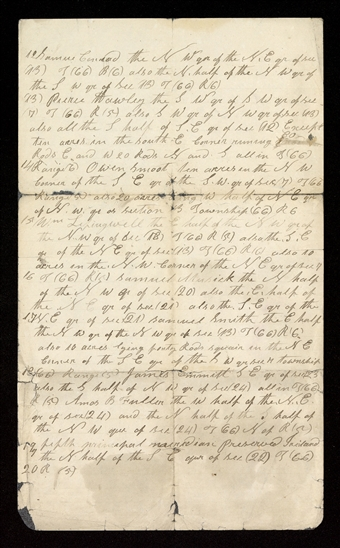# Lands Deeded, after 26 June 1839

• Source Note
Page<​12​> Samue[l] Conrad the N W qr of the. N. E qr of sec (13) T (66) R (6) also the N. half of the N W qr of the S w qr of Sec (13) T (66) R (6)
(13) the S. W qr of S W qr of sec (7) T (66) R (5) also S W qr of N w qr of. sec (13) also all the S half of S. E qr of sec (12) Except ten acres in the south E Corner run[n]ing 8 rods <​80​> Rods E and W 20 Rods N and S all in T (66) <​14​> Range (6) [Abraham] Owen Smoot ten acres in the N W Corner of the S E qr of the S. W. qr of sec (7) T (66) Range (5) also 20 acres ◊◊ng W half of N E qr of N. w. qr of section 13 Township (66) R 6 <​15​> Wm Lepingwell the E half of the N W qr of the N. w qr of sec (18) T (66) R (5) also the. S. E qr of the N E qr of sec (13) T (66) R (6) also 10 acres in the. N. W. Corner of the N E qr of sec 7 <​16​> T (66) R (5) the N half of the N w qr of sec (20) also the. E. half of the N E qr of sec (21) also the. S. E qr of the <​17​> N. E qr of sec (21) the E half the N w qr of the N w qr of sec (13) T (66) R (6) also 10 acres lying forty Rods squair in the N E Corner of the S E qr of the S w qr sec 17 township <​18​> (66) Range (5) S E qr of sec (23) also the S half of the N W qr of sec (24) also in T (66) R (5) the W half of the. N. E. qr of sec (24) and the N half of the S half of the N W qur of sec (24) T (66) N of R (5) <​19​> <​of​> fifth princapal maridian preserved Iriland the N half of the S E qur of sec (22) T (66) <​20​> R (5) [p. ]
12 Samuel Conrad the N W qr of the. N. E qr of sec (13) T (66) R (6) also the N. half of the N W qr of the S w qr of Sec (13) T (66) R (6)
(13) the S. W qr of S W qr of sec (7) T (66) R (5) also S W qr of N w qr of. sec (13) also all the S half of S. E qr of sec (12) Except ten acres in the south E Corner running 80 Rods E and W 20 Rods N and S all in T (66) 14 Range (6) [Abraham] Owen Smoot ten acres in the N W Corner of the S E qr of the S. W. qr of sec (7) T (66) Range (5) also 20 acres ng W half of N E qr of N. w. qr of section 13 Township (66) R 6 15 Wm Lepingwell the E half of the N W qr of the N. w qr of sec (18) T (66) R (5) also the. S. E qr of the N E qr of sec (13) T (66) R (6) also 10 acres in the. N. W. Corner of the N E qr of sec 7 16 T (66) R (5) the N half of the N w qr of sec (20) also the. E. half of the N E qr of sec (21) also the. S. E qr of the 17 N. E qr of sec (21) the E half the N w qr of the N w qr of sec (13) T (66) R (6) also 10 acres lying forty Rods squair in the N E Corner of the S E qr of the S w qr sec 17 township 18 (66) Range (5) S E qr of sec (23) also the S half of the N W qr of sec (24) also in T (66) R (5) the W half of the. N. E. qr of sec (24) and the N half of the S half of the N W qur of sec (24) T (66) N of R (5) 19 of fifth princapal maridian preserved Iriland the N half of the S E qur of sec (22) T (66) 20 R (5) [p. ]
Page